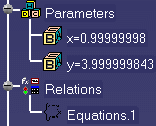# Solving a Set of EquationsThis task explains how to solve a set of equations using the operators and functions of the knowledgeware language. This scenario can be run from any document.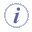In a set of equations, the semi-colon (;) is used as a separator. Note that the equations set capabilities require the Knowledge Advisor product.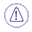Note that a KWA license is required to work with sets of equations.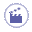1. Create two real type parameters x and y. Both parameters are intended to be used as variables in a set of equations.

2. Access the Knowledge Advisor workbench. Click the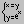icon. In the first dialog box which is displayed, enter the name of the relation, a comment and a destination. Then click OK. The Set Of Equations editor is displayed.

3. Enter the set of equations below into the edition box:
```y - 2 *sqrt(x) ==2; y - 4 *x*x == 0`````` ```Now, your editor looks something like this: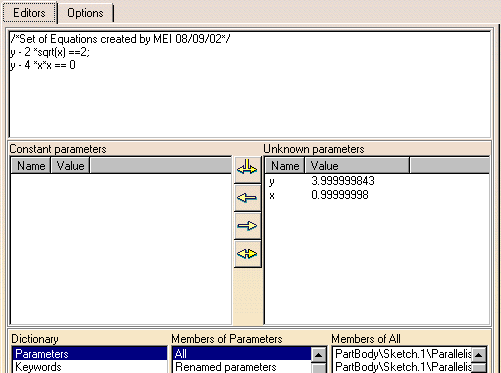The value of each parameter is displayed first in the Unknown parameters field, then in the specification tree (see below).